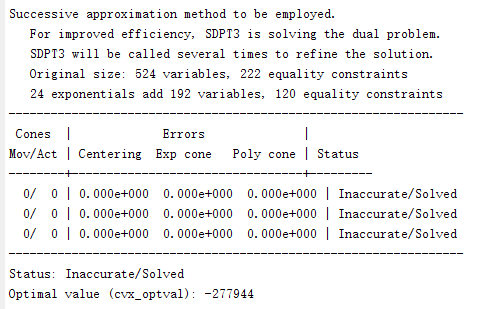# Optimal value (cvx_optval) cvx obtained is not the same as the result obtained by the optimal solution

Dear everyone,

My code is followed,

clear;
clear all;
k = 8; % The number of user in a small cell
PL = 0;
r = ones(1,k);

%Simulation parameters
n = 0.3; % shared fraction, 0<=n<=1
N0 = 1e-5; % noise power spectral density（dBm/Hz）
%N0 = power(10,N0/10)/1000; % Converted to w/Hz
Wul = 1e6; Wdl = 1e6; % The uplink and downlink bandwidth (Hz)
Pumax = 100; Pdmax = 1000; % The uplink and downlink power（w）
FC = 10^8; % The cloudlet server processing capacity（CPU cycles/s）
luk = 1.7810^-6; % The amount of energy spent by the mobile device to extract each bit of offloaded data from the video source（J/bit）
ldk = 0.625; % Parameter that captures the mobile receiving energy expenditure per second in the downlink（J/s）
BIk = 1e4
ones(1,k); % Input bits from each user k to the cloudlet in the uplink (1Mbits)
BOk = 1e4ones(1,k); % Out bits from the cloudlet to each user k in the downlink (1Mbits)
%Vk = 2640
BIk; % Processing the input BIk by executing Vk CPU cycles at the cloudlet (CPU cycles)
%Vs = nVk; % Shared CPU cycles
BOs = n
BOk; % Shared output bits that delivered to all users
BIs = n*BIk; % Shared input bits that can be sent by any of the users

%Initialize the z = [Pul,Bls,f,Pdl,PdM,TuS,TdS]
Pulk0 = Pumaxrand(1,k); % The uplink power（w）of user k
%Pdlk0 = Pdmax/k
ones(1,k); % The downlink power（w）to user k
Pdlk0 = rand(1,k);
Pdlk0 = Pdlk0/(sum(Pdlk0))Pdmax;
PdlM0 = Pdmax
rand(1,1); % Transmit power (w) for multicasting
%BIsk0 = BIs/k.*ones(1,k); % A fraction of BIsk bits of the BIs shared bits transmited by user k
BIsk0 = rand(1,k);
BIsk0 = BIsk0/sum(BIsk0).*BIs;
fk0 = rand(1,k);
fk0 = fk0/(sum(fk0));
fs0 = rand(1,1); % Fractions of the processing power FC assigned to run the Vk CPU cycles exclusively for user k and the VS shared CPU cycles
f0 = [fk0,fs0];

Vk = 2640*(BIk-BIsk0+BIs);
Vs = 2640*BIs;

Rulk0 = (Wul/k)log2(1+PU0/(N0Wul/k)); % Transmitting rate of user k in the uplink
PD0 = r.
Rdlk0 = (Wdl/k)log2(1+PD0/(N0Wdl/k)); % Transmitting rate to user k in the downlink
PM0 = r.(PdlM0-PL); % Multicasting power after rayleigh fading
RdlMk0 = Wdl
log2(1+PM0/(N0*Wdl)); % Transmitting rate to users for multicasting
TuS0 = max(BIsk0./Rulk0); % The time to complete the shared uplink transmissions (s)
TdS0 = max(BOs./RdlMk0); % The downlink transmission time to multicast BOs bits
Tmax = 2; % Maximum latency constraint（0.15s/0.05s）
z0 = [Pulk0,BIsk0,f0,Pdlk0,PdlM0,TuS0,TdS0];
alpha = 10^-5; epsilong = 10^-5; delta0 = rand; % Accuracy parameters

``````%cvx_solver SeDuMi
%cvx_solver ecos
%cvx_solver mosek
cvx_begin
variables Pulk(1,k) BIsk(1,k) fk(1,k) fs Pdlk(1,k) PdlM TuS TdS;
f = [fk,fs];
PU = r.*(Pulk-PL);
Rulk = (Wul/k)*log(1+PU/(N0*Wul/k))/log(2);
PD = r.*(Pdlk-PL);
Rdlk = (Wdl/k)*log(1+PD/(N0*Wdl/k))/log(2);
PM = r.*(PdlM-PL);
RdlMk = Wdl*log(1+PM/(N0*Wdl))/log(2);

%The overall downlink mobile energy consumption for user k
Edlk = ((BOk-BOs).*inv_pos(Rdlk)+(BOs.*inv_pos(RdlMk)))*ldk;

%Mobile energy consumption of uplink transmission
z = [Pulk,BIsk,f,Pdlk,PdlM,TuS,TdS];
``````

% E = (z-z0)diag(z0)(z-z0)’;
e1 = Pulk0.(BIsk0+(BIk-BIsk0)).inv_pos(Rulk);
e2 = Pulk0.
(BIsk+(BIk-BIsk0))./Rulk0;
e3 = Pulk.
(BIsk0+(BIk-BIsk0))./Rulk0;
e4 = luk*(BIsk+(BIk-BIsk0));
Eulk = e1+e2+e3+(z-z0)diag(z0)(z-z0)’+e4;

``````    % Rewrite the latency constraints C.2
g1 = square_pos(BIsk+inv_pos(Rulk));
g2 = Rulk0.*Rulk0;
g3 = (g1-BIsk0.^2-1./g2);
g4 = BIsk0.*(BIsk-BIsk0);
g5 = (r.*Pulk0)/(N0*Wul/k);
g6 = (1+g5).*(Rulk0.^4);
g7 = g4-(Rulk0./g6).*(inv_pos(Rulk)-1./Rulk0);
gk = 1/2*g3-g7;

minimize(sum(Eulk+Edlk))
subject to
(BIk - BIsk0).*inv_pos(Rulk)+(Vk-Vs)/FC.*inv_pos(fk)+Vs/FC*inv_pos(fs)+(BOk-BOs).*inv_pos(Rdlk) <= Tmax-TuS-TdS;   % Constraint C.1
gk < TuS;                            % Constraint C.2
BOs.*inv_pos(RdlMk) <= TdS;           % Constraint C.3
sum(fk) == 1;                         % Constraint C.4
0 < fk;
0 <= fs < 1;
sum(BIsk) == BIs;                     % Constraint C.5
sum(Pdlk) <= Pdmax;                   % Constraint C.6
10^-5 < Pdlk <= Pdmax;
10^-5 < PdlM <= Pdmax;
10^-5 < Pulk <= Pumax;
0 <= TuS <= Tmax;
0 <= TdS <= Tmax;
0 <= BIsk <= BIs;
cvx_end
``````

In my code, the object function is min(sum(Eulk+Edlk)), and Eulk = e1+e2+e3+(z-z0)diag(z0)(z-z0)’+e4, Edlk = ((BOk-BOs).*inv_pos(Rdlk)+(BOs.*inv_pos(RdlMk)))*ldk. The result I got followed:The optimal value obtained by cvx directly is not the sane as the result calculated by optimal solution.
Why did the result happen?

Thank you.I “solved” this same problem using CVX 2.1 and SDPT3 with result “Inaccurate.Solved”, cvx_optval = -146276. Note however that because rand is used, my random numbers, and therefore problem instantiation, is likely different than yours.

That said, sum(Eulk+Edlk) comes out to -146276, matching my cvx_optval. I believe what you encountering is a feature not a bug of CVX. See my answers at The return value in the process of cvx is Incorrect . Also see my answers and mcg’s answers at Abnormal result of lambda_max function .

Hi Mark, I have read the answers you provided. But I still have some troubles.

While I used CVX to obtained a cvx_optval, I got the value of cvx variables. Does this means that we can get the optimal value equal to cvx_optval by using the value of cvx variables?

In my code, the value of cvx variables are positive number after by CVX. According to the formula, the cvx_optval should be positive. But I got a negative number. Does my code have some errors?